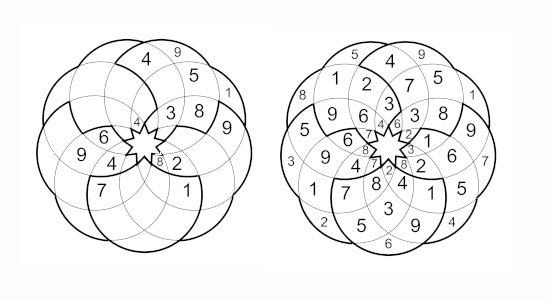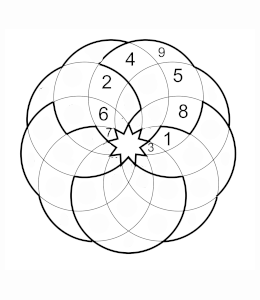Rules of Sudoku Rosace

## Sudoku RosaceThe Rosace Sudoku is made of circles. Instead of having straight lines this puzzles have curves this is why the cells do not have the same size. The puzzle has nine circles that overlap. The rules are the same in each region put numbers from 1 to 9. As the puzzle is made of curves the distinction between a row and a column is difficult, I will call it arcs. Therefore each arc must have the numbers from 1 to 9.

This is a region filled with numbers from 1 to 9 ( the puzzle has 5 regions).This is an arc filled with numbers from 1 to 9 ( the puzzle has 5 regions) each cell (except the ones at the extremities -ex where is the nine) belong to two arcs. The puzzles has 9 arcs.Because we are used to playing with straight lines, the curving lines add a new challenge. Try it you will be pleased by this puzzle.